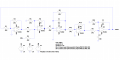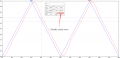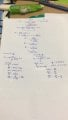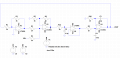richard_lai

Joined Nov 21, 2019
24
I'm doing my project on modern control system and had already design my circuit as below. The problem I'm facing is to eliminate the steady steady error. The steady state error is the second picture. I know that this is a first order system and in order to eliminate the steady state error, I need to add another integrator circuit. But I dont know how to calculate the values and also which type of integrator to use, i know there are two types which is inverting and non inverting but i dont know how to choose between these two types. The requirements is to eliminate the error without changing the original circuit which is can only add another circuit outside the circuit below and with the output still monitored. If a circuit need to be added, where is it need to be? After the Vin or need to have a summing amplifier with the input with some feedback?RBR1317

Joined Nov 13, 2010
676
I'm confused here. You have defined the steady-state error at a point where the system is not in a steady state; i.e., at a point where the input stimulus (Vin) is in a transient state. Does the final value theorem not apply anymore?

richard_lai

Joined Nov 21, 2019
24
I'm confused here. You have defined the steady-state error at a point where the system is not in a steady state; i.e., at a point where the input stimulus (Vin) is in a transient state. Does the final value theorem not apply anymore?
My input is a ramp input and after calculation, the steady state error is same as the one simulated as well as on the real circuit. We are asked to add circuits such as integrator to eliminate the errors.

crutschow

Joined Mar 14, 2008
28,516
If R8 is removed, you will have a integrator in the loop.
But steady-state error is normally considered to be when the loop is steady (no change in voltage), not when it is varying, as you show.

SteveSh

Joined Nov 5, 2019
105
Change your input from a saw tooth to a square wave and see what you get.

MrAl

Joined Jun 17, 2014
8,607
I'm doing my project on modern control system and had already design my circuit as below. The problem I'm facing is to eliminate the steady steady error. The steady state error is the second picture. I know that this is a first order system and in order to eliminate the steady state error, I need to add another integrator circuit. But I dont know how to calculate the values and also which type of integrator to use, i know there are two types which is inverting and non inverting but i dont know how to choose between these two types. The requirements is to eliminate the error without changing the original circuit which is can only add another circuit outside the circuit below and with the output still monitored. If a circuit need to be added, where is it need to be? After the Vin or need to have a summing amplifier with the input with some feedback?
View attachment 192155
View attachment 192156
Hello,

Can we see your calculation for the transfer function?
You can factor out one true integrator from that last stage so i would have guessed a small steady state error, or a very very large one, but let's see your calculation.

richard_lai

Joined Nov 21, 2019
24
If R8 is removed, you will have a integrator in the loop.
But steady-state error is normally considered to be when the loop is steady (no change in voltage), not when it is varying, as you show.
Change your input from a saw tooth to a square wave and see what you get.
My assignment is ask in the response to ramp input so need to fix to this waveform.

richard_lai

Joined Nov 21, 2019
24
Hello,

Can we see your calculation for the transfer function?
You can factor out one true integrator from that last stage so i would have guessed a small steady state error, or a very very large one, but let's see your calculation.
Yeap sure as attatched below. I saw u answer one of my classmates question on designing. The question are same but im finding method to eliminate the steady state error and i already get the circuit. My calculation are as below.My circuit are changed to this to use less opamp and simplify the circuit.MrAl

Joined Jun 17, 2014
8,607
Yeap sure as attatched below. I saw u answer one of my classmates question on designing. The question are same but im finding method to eliminate the steady state error and i already get the circuit. My calculation are as below.View attachment 192230

My circuit are changed to this to use less opamp and simplify the circuit.
View attachment 192231
Hi,

Ok so do you still have the problem or no?

richard_lai

Joined Nov 21, 2019
24
Hi,

Ok so do you still have the problem or no?
The circuit worked for mine but now another task is we are asked to eliminate the steady state error.

crutschow

Joined Mar 14, 2008
28,516
What exactly does that mean?

richard_lai

Joined Nov 21, 2019
24
What exactly does that mean?
By measuring the circuit through oscilloscope in response to a ramp input, there will have a steady state error of 360mV (could refer to #1 attachment. I need add some more circuit to eliminate the error. I just know that need to let it become second order as for ramp response second order onward wont have error. But i dont know what circuit to add and what value of components(calculation).

MrAl

Joined Jun 17, 2014
8,607
The circuit worked for mine but now another task is we are asked to eliminate the steady state error.
Hello again,

Is this in theory only or for a practical circuit?
I ask because in a practical circuit there could be some error due to the op amp package input offset. If the offset is integrated, it becomes larger.
I also ask because in the theoretical case i did not find any steady state error except for maybe a very small amount, could that be the only error?

Can you do another plot of your new circuit?
By changing circuits we have to more or less start over.

richard_lai

Joined Nov 21, 2019
24
Hello again,

Is this in theory only or for a practical circuit?
I ask because in a practical circuit there could be some error due to the op amp package input offset. If the offset is integrated, it becomes larger.
I also ask because i did not find any steady state error except for maybe a very small amount, could that be the only error?
The graph i attatch is theory but the practical circuit also has same error and also same value

MrAl

Joined Jun 17, 2014
8,607
What exactly does that mean?
Hi,

This is a fairly common problem in control systems especially feed forward only systems.
If for example you are expecting a 0.000v output and you get a 0.010v output then the steady state error is 0.010 volts. If you are expecting a 1.000 volt output and you get a 0.990 volt output then the steady state error is -0.010 volts.

Last edited:

MrAl

Joined Jun 17, 2014
8,607
The graph i attatch is theory but the practical circuit also has same error and also same value

Can you do a plot of your NEW circuit?
I dont see much error so i was wondering how much you think the error is, with your plot like you did last time for the older circuit.

Another issue we might want to address is the problem of using the technique of pole/zero cancelation. I would ask if your instructor allows that as i see one of your stages depends highly on the accuracy of that implementation method. We can wait till much later if you like.
This also brings up the question of if you are doing a practical circuit or you just have to adjust the system in theory only (which often disregards some little things like input offset).

richard_lai

Joined Nov 21, 2019
24
Can you do a plot of your NEW circuit?
I dont see much error so i was wondering how much you think the error is, with your plot like you did last time for the older circuit.

Another issue we might want to address is the problem of using the technique of pole/zero cancelation. I would ask if your instructor allows that as i see one of your stages depends highly on the accuracy of that implementation method. We can wait till much later if you like.
Its the same error. I just change the first order filter from inverting to non inverting to use less op amps. the behaviour all are the same

MrAl

Joined Jun 17, 2014
8,607
Its the same error. I just change the first order filter from inverting to non inverting to use less op amps. the behaviour all are the same
Hello again,

I took another look at this and it looks like what you are talking about might better be described as an offset. To eliminate offset you have to use either an adder or subtractor.
If you have say 0.3v offset then you subtract 0.3v offset.
If the offset is related to the input then you have to use a special circuit to calculate the offset and apply that to some place in the circuit.
But what methods have you been taught so far? That would help determine what way they want you to do it.